# Java Program to check Palindrome string using Recursion

In this tutorial you will learn about the Java Program to check Palindrome string using Recursion and its application with practical example.

## Java Program to check Palindrome string using Recursion

In this tutorial, we will learn to create a Java program that will check Palindrome string using Recursion using Java programming.

## Prerequisites

Before starting with this tutorial, we assume that you are the best aware of the following Java programming topics:

• Operators in Java Programming.
• Basic Input and Output function in Java Programming.
• Basic Java programming.
• For loop in Java programming.
• Conditional Statements in Java programming.
• Arithmetic operations in Java Programming.

## What is a palindrome string/number?

The Palindrome string/number means a string/number that is the same when we read it from forward and reverse.

For Example 1:- AddA is a palindrome string that is the same when we write it in reverse order, 1221

Example 2:- 1221 this is a palindrome number that is the same when we write it in reverse order, 1221.

Example 3:- 123 this is not a palindrome number, it is not the same when we write it in reverse order, 321.

## Program to Check Whether a String is Palindrome or Not:-

To check whether a number is a Palindrome string or not. In today’s Java program, we will write code for checking. First, we will take a string of input from the user. Then we will pass it into the program to Check Whether a String is Palindrome or Not.

With the help of the below program, we can Check the Palindrome string or Not.

## Output:-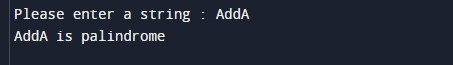In the above program, we have first initialized the required variable.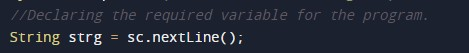• strg = it will hold the string value of the input for the program.

Input message for the user for the string value.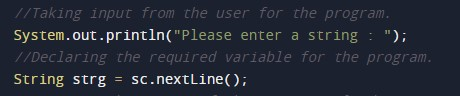Program Logic Code to check the string is palindrome or not.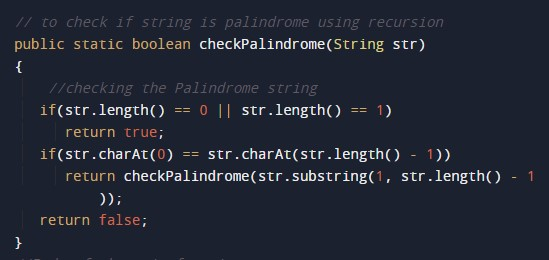Printing output Palindrome number or not.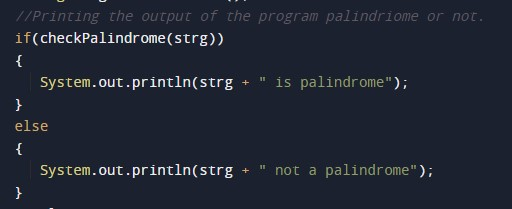In this tutorial we have learn about the Java Program to check Palindrome string using Recursion and its application with practical example. I hope you will like this tutorial.